-->

# Most Scoring Topics in JEE Main: Maths

## Why Mathematics is considered the most scoring subject in JEE Main

IIT JEE is considered to be one of the toughest examinations at the undergraduate level. Although, all the subjects of this examination have equal weightage in terms of marks but the subject which scares most of the students is Mathematics. Even the sharpest of students end up making the silliest of mistakes when it comes to Mathematics.

The examination is scheduled to be held on April 15-16, 2018 which leaves the students with approximately 1 month to excel in their preparation. Candidates can download JEE Main admit card from the official website.

While Maths is scoring and lengthy section, it requires rigorous practice and persistence to score well. Looking at the level of difficulty for the examination, wouldn’t it be beneficial if you had a list of topics which were easy to score in? Fret now, we have just the same. The below mentioned topics are important yet scoring at the same time!

## Trigonometry :

Generally, you will not find hardcore pure trigonometry questions in JEE Mains. Mostly, questions from inverse trigonometry and trigonometric equations are asked in the exam. They are quite easy to do if you remember the trigonometric formulas. In some questions, one can guess the answer by simply looking at the options. This is one area which can definitely benefit you. One can expect easy questions from this area.

## Algebra :

Quadratic equations and matrices are the most widely covered areas in algebra, asked in the examination. Usually the exam consists of a lot of easy questions and also has high weightage in JEE Mains. From matrices, you can expect easy passage type questions.

Multiple questions can be attempted through a single passage. Apart from matrices, quadratic equations are also one of the most favorite topics of students aspiring for JEE. One should devote a lot of time to this topic as it is easy scoring. But, you may also expect some standard questions from this area so a strong practice is highly recommended.

## Permutations and combinations :

This is a topic which is easy in nature with very few formulas but it is an unpredictable topic. Sometimes, you can expect lot of questions from this area and sometimes very less questions from this area. Mostly, more or less the questions will be similar to the ones asked in previous years.

## Probability :

It is a logical area from where you can expect easy questions. However, the questions are conceptual based and not based on formulae’s so you need to focus more on the logic part.

## Co-ordinate geometry :

In coordinate geometry, most of the questions appear from straight lines and circles. These topics also involve analytical skill as there are not much formula’s in it. The best part is that this chapter also appears in class 10th and most of the questions asked from this area are from basics. So, getting a strong hold on fundamentals would help you in scoring well in this area.

## Differentiation and Integration Calculus :

These topics scare students the most because they have lot of formulas in them. However, once you learn the formulas and start practicing it is also one of the most interesting areas in Maths. Under differentiation, Limits and continuity is one of the easiest and scoring topics. The whole topic is based on only one simple “L-Hospitals” rule which makes it very easy and scoring.

In integration, which is also called reverse of differentiation, there are lot of formulas. But, one easy area in the same is definite integrals with very few formulas, making it easier to learn and practice questions based on this topic. The Physics section also has a lot of questions based on Integration and thus, one must focus on it.

## Statistics :

Statistics is another easy area where you just need to memorize basic formulas. Questions are based on mean, median, mode etc. This is a complete formula based area and hence, you won’t get any tricky questions. Increase your score by simply applying the formulas.

## Vectors & 3D:

Last but not the least, vectors and 3D is an area which appears in the ending chapters of the curriculum. This topic is very easy and scoring. A lot of questions are asked from this section. Problems are generally easy in nature and this topic is an extension of coordinate geometry so, if you are thorough with concepts of coordinate geometry vectors and 3d would be easy too. Planes is an easy area in vectors and highly scoring too. However, you can expect direct questions from this area so be clear with the concepts.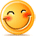:)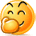:-)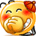:))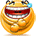=))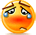:(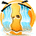:-(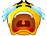:((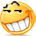:d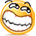:-d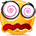@-)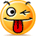:p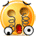:o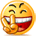:>)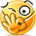(o)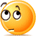[-(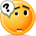:-?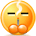(p)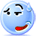:-s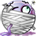(m)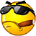8-)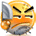:-t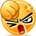:-b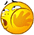b-(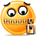:-#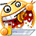=p~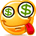:-\$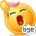(b)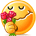(f)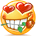x-)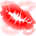(k)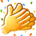(h)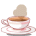(c)cheer